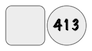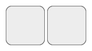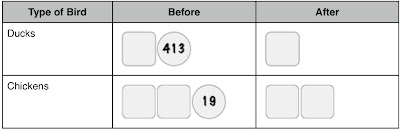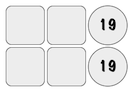## Sunday, May 15, 2011

### Pictorial Ways of Solving Algebraic Problems

A farmer had twice as many ducks as chickens. After the farmer sold 413 ducks and 19 chickens died, he has half as many ducks as chickens. How many ducks does he have now?

I have been studying ways to solve a simple problem in algebra pictorially rather than the traditional methods as I think through how to teach Pamela how to do this one day. Since I know many folks scared off by high school math occasionally pop into my blog, I was wondering if this makes more sense to you than the traditional method which I list at the end of this post. This pictorial method incorporates two strategies: (1) working backward from the answer and (2) incorporating the techniques taught in the first four chapters of Jacob's Elementary Algebra. Pamela has been using an empty box for the unknown for years, based on how Making Math Meaningful teaches whole-part thinking.

Ducks
We want to know the number of ducks the farmer has now, which is our unknown, represented by the empty box.We know that the farmer used to have 413 more ducks than he has now because he sold that many. So, at the beginning of the problem, the farmer had whatever number he has now and the 413 he sold.Chickens
We want to know the number of chickens the farmer has now. We know that he has half as many ducks as he does chickens. If he has 8 ducks, he would have 16 chickens. That means that the number of chickens is double the number of ducks. Whatever the number of ducks is now, the number of chickens is twice the amount.We know that the farmer used to have 19 more chickens than he has now because that many chickens died. So, at the beginning of the problem, the farmer had whatever number of chickens he has now and the 19 that died.Summarize the Quantity of Ducks and Chickens Before and AfterWe were given one more relationship: at the beginning of the problem, he had twice as many ducks as chickens. If he had 8 ducks, then he had 4 chickens. That means the number of ducks at the beginning was twice the number of chickens. Twice the number of chickens at the beginning would be double of what is in the table, orWe can rewrite twice the number of chickens as,The number of ducks at the beginning of the problem is the same as double the number of chickens,We can decompose 413 in a way to help us see the answer:
413 = 375 + 38 = 125 + 125 + 125 + 38That means 125 goes into the empty box, which is the number of ducks that the farmer has now.

To check our work, we can plug 125 into the empty boxes into the table. The number of chickens now is twice what was in the empty box, 2 x 125 or 250. The number of ducks at the beginning was the empty box and 413, 125 + 413 or 538. The number of chickens at the beginning was the twice the empty box and 19, or 2x125 + 19 or 269.

Does this make sense? At the beginning of the problem, the number of ducks (538) is twice the number of chickens (269). At the end of the problem, the number of ducks (125) is half the number of chickens (25). All relationships make sense!

Other Methods:
I found this problem at a Singapore Math blog, which offers multiple problem solving strategies that are more pictorial than the traditional method. The author linked to a more thorough explanation of Singapore's model method that you might enjoy.

Let c be the number of chickens and d be the number of ducks at the beginning of the problem. Since there are twice as many ducks as there are chickens at the beginning,
d = 2c

Since the farmer sold 413 ducks, the number of ducks at the end of the problem is the expression,
d - 413

Since 19 chickens died, the number of chickens at the end of the problem is the expression,
c - 19

We also know that now there are half as many ducks as chickens, so we can write an equation for this relationship:
d - 413 = ½(c - 19)

Substituting the first relationship between ducks and chicks, we can now solve for the number of chickens at the beginning of the problem.
2c - 413 = ½(c - 19)

2(2c - 413) = 2[½(c - 19)]

4c - 826 = c - 19

4c - 826 - c = c - 19 - c

3c - 826 + 826 = -19 + 826

3c = 807

c = 269

If the number of the chickens at the beginning of the problem was 269, the number of ducks was twice that, or 538. If the number of ducks at the beginning of the problems was 538, the number of ducks at the end is 413 less than that, or 125.

To check our work, if the number of chickens at the beginning of the problem was 269, the number of chickens at the end is 19 less than that, or 250. Since 250 is twice 125, the number of chickens is now twice the number of ducks.

JEMD1966 said...

Tammy, the "pasted graphics" are not coming through whne I try to view you blog. Any suggestions? I'm super interested in this topic because this is the year I plan to make a major change in Tim's math curriculum...thanks so much for all that you do!Amy O'Connor said...

Tammy,
I need a little help here - in the beginning the problem states the farmer has twice as many ducks as chickens, so more ducks than chickens but the solution shows he has more chickens than ducks to start with - what am i missing?
-Amy

walking said...

JEMD, thanks for letting me know about the graphics glitch. You should see the pictures now.

Amy, thank you for being so careful in your reading. I have contacted the math blog that was the source of this problem. The entire problem is written in various forms of present tense, even events that occurred in the past. It may be the style in which Singapore math problems are written. I rewrote my version to have a more clear tense.